# Python Operators

## Introduction

In this article, I'll take you through the type of operators we do use in Python. These are:

## Operators in Python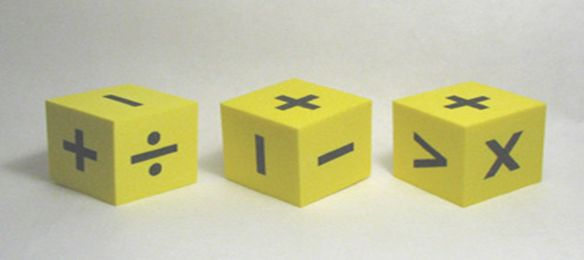• Arithmetic Operators
• Special Operators
• Comparison Operators
• Bitwise Operators
• Assignment Operators
• Identity Operators

## Arithmetic Operators

For performing the base mathematical operations or some other type of math-related functionality we use Arithmetic Operators.

Let's take a close look at all these operators.

 Operator Description Example Addition Adds both operands together z=x+y Subtraction Subtracts the right-side operand from the left-side operand z = x – y Multiplication Multiplies both operands together z = x * y Division Divides the left-side operand with the right-side operand z = x / y Modulus It takes a modulus using two operands and assigns the result to the left z = x % y

Flow Chart | Arithmetic Operators

Here's a flow chart showing how the operational flow goes. Let's have a close look at this.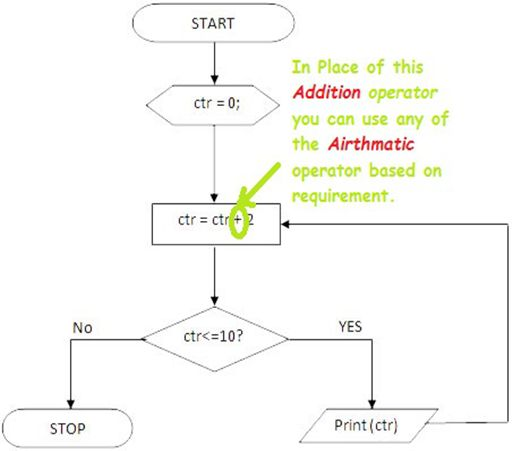(If you need examples for every operator then let me know and I'll update it.)

## Special Operators

Actually these are not special operators, these are a subset of mathematical operators, that does the same functionality as general mathematical operators do.

Let's take a close look at all these operators.

 Operator Description Example Exponent Does exponential operations x ** y = x ^ y Floor Division Takes the result of the quotient and rejects the mantissa 5 // 2 = 2

(If you need examples for every operator then let me know and I'll update it.)

## Comparison Operators

The comparison operators are used to compare the values of both sides of the operators and do specific operations based on them.

Let's take a close look at all these operators.

 Operator Description Example == Compares both the left and right operands and depending on the result, displays either True or False (2 == 3)false > Compares the left operand with the right operand and depending on the result, (greater) displays either True or False (3 > 2)true < Compares the left operand with the right operand and depending on the result, (left) displays either True or False (3 < 2)true != If the values of the two operands aren't equal, then displays the condition accordingly (3 != 2)true <> If the values of both operands aren't equal then the condition becomes true (3 <> 2) true >= If the value of the left operand is greater than the right-side operand, then the condition becomes true (3 >= 2)true <= If the value of the left operand is greater than the right-side operand, then the condition becomes true (3 <= 2)false

Flow Chart | Comparision Operators

Here's a flow chart showing how the operational flow goes. Let's have a close look at this.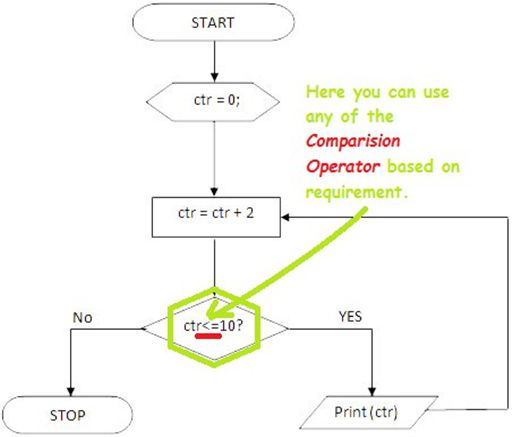(If you need examples for every operator then let me know and I'll update it.)

## Bitwise Operators

Bitwise operators are bit-oriented operators. Here bit-oriented means that these operators work on the bits and do the action bit by bit.

Let's take a close look at all these operators.

 Operator Description Example &(Binary AND) It copies a bit to the result if it exists in both operands (x & y) |(Binary OR) It copies a bit if it exists in either operand (x | y) ~(Binary One's Complement) It's used for flipping bits (~x) ^(Binary XOR) It copies the bit if it is set in one operand but not both (x ^ y) <<(Binary Left Shift) It shifts the value to the left specified by the right operand x << 2 >>(Binary Right Shift) It shifts the value to the right specified by the right operand x >> 3

Flow Chart | Bitwise Operators

Here's a flow chart showing how the operational flow goes. Let's have a close look at this.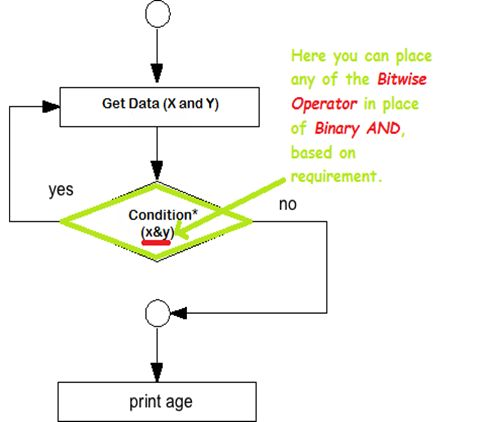(If you need examples for every operator then let me know and I'll update it.).

## Assignment Operators

As the name suggests, assignment operators are used for assigning values, for example to a variable when performing certain operations.

Let's take a close look at all these operators.

 Operator Description Example = It assigns a value from the right-side operand to the left side z = x + y(value of x + y -> z ) += Adds and it adds the right operand to the left operand and assigns the result to the left operand z += x(z = z + x) -= Subtracts and it subtracts the right operand from the left operand and assigns the result to left operand z -= x(z = z - x) *= Multiplies and it multiplies the right operand with the left operand and assigns the result to the left operand z *= x(z = z * x) /= Divides and it divides the left operand by the right operand and assigns the result to the left operand z /= x(z = z / x) %= Takes the modulus using the two operands and assigns the result to the left operand z %= x(z = z % x)

Flow Chart | Assignment Operators

Here's a flow chart showing how the operational flow goes. Let's have a close look at this.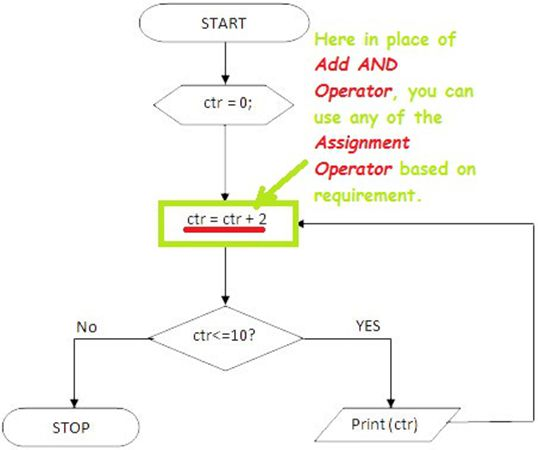(If you need examples for every operator then let me know and I'll update it.).

## Identity Operators

Identity operators are used to comparing the memory locations of two objects (compare the locations).

Let's take a close look at all these operators.

 operator Description Example is Evaluates whether the values on either side are equal or not and acts (if true) accordingly. If x is yresults True Is NOT Evaluates whether the values on either side are equal or not and acts (if false) accordingly. If x is not yresult False

Flow Chart | Identity Operators

Here's a flow chart showing how the operational flow goes. Let's have a close look at this.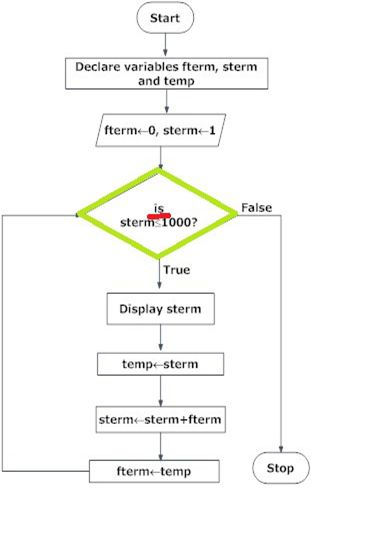## Conclusion

In the next chapter, we will talk about Python Data Types.
Next » Python Data Types